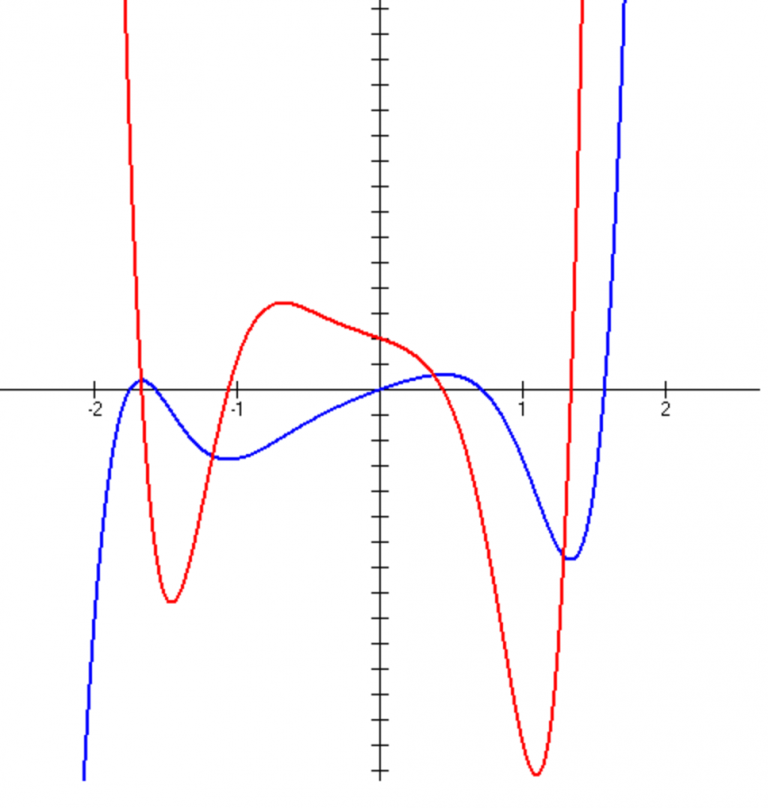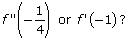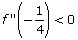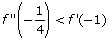# Problem 9: The Graph of a Derivative

2011-2012 Derivative Graph In the below graph, two functions are pictured, f(x) and its derivative, but I can’t seem to tell which is which. According to those graphs, which is greater: Solution: You might have noticed that the red function has an even degree whereas the blue has an odd degree. Why? An even-degreed function’s ends will … Continue reading "Problem 9: The Graph of a Derivative"## 2011-2012 Derivative Graph

In the below graph, two functions are pictured, f(x) and its derivative, but I can’t seem to tell which is which.According to those graphs, which is greater:### Solution:

You might have noticed that the red function has an even degree whereas the blue has an odd degree. Why? An even-degreed function’s ends will either both point up or both point down (here they both point up at the edges of the graph), whereas a function with an odd degree has ends that go in opposite directions (like the blue graph which goes down to the left but up to the right). Even though this is interesting, it is not enough to answer the question.

You may also say that the red graph is definitely a lesser degree because it has a fewer number of x-intercepts (4) than the blue graph (5), but this, too, is not sufficient information to answer the question correctly. It is true that the blue graph is f(x) and the red is f ‘(x), but for different reasons.

Here are two acceptable justifications for identifying the blue graph as f(x):

• Whenever the blue graph has a relative max or min (hilltop or valley bottom, respectively), the red graph has an x-intercept; remember that the derivative of a function is either 0 or undefined when the original function has a relative extrema point
• Whenever the blue graph is increasing, the red graph is positive (i.e., above the x-axis), and when the blue graph is decreasing, the red is negative

Now that we’ve got that straight, let’s get down to answering the question. You have no idea what the exact function values are, but it turns out that you don’t really need to know them! Since the red graph is decreasing at x = –1/4, then its derivative must be negative there (for the same reason that the second bullet above is true).What about f (–1)? Because the red graph represents f ‘(x), it’s easy to see that the red graph is just above the x-axis when x = –1. Therefore, f ‘(–1) > 0.

Back to the original question, which asks you to compare two values. You now know that one of those values is positive and the other is negative, so the positive value must be greater.The video may take a few seconds to load.Having trouble Viewing Video content? Some browsers do not support this version – Try a different browser.